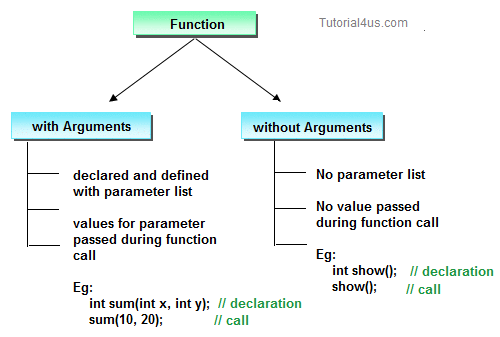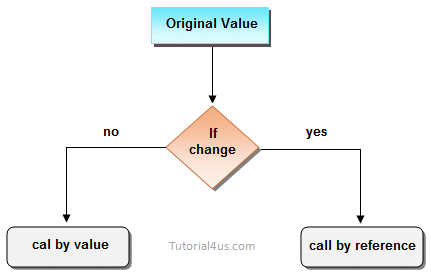# Function Arguments in C++

## Call by Value and Call by Reference in C++

On the basis of arguments there are two types of function are available in C++ language, they are;• With argument
• Without argument

If a function take any arguments, it must declare variables that accept the values as a arguments. These variables are called the formal parameters of the function. There are two ways to pass value or data to function in C++ language which is given below;

• call by value
• call by reference## Call by value

In call by value, original value can not be changed or modified. In call by value, when you passed value to the function it is locally stored by the function parameter in stack memory location. If you change the value of function parameter, it is changed for the current function only but it not change the value of variable inside the caller function such as main().

## Call by value

```
#include<iostream.h>
#include<conio.h>

void swap(int a, int b)
{
int temp;
temp=a;
a=b;
b=temp;
}

void main()
{
int a=100, b=200;
clrscr();
swap(a, b);  // passing value to function
cout<<"Value of a"<<a;
cout<<"Value of b"<<b;
getch();
}
```

```
Value of a: 200
Value of b: 100
```

## Call by reference

In call by reference, original value is changed or modified because we pass reference (address). Here, address of the value is passed in the function, so actual and formal arguments shares the same address space. Hence, any value changed inside the function, is reflected inside as well as outside the function.

## Example Call by reference

```
#include<iostream.h>
#include<conio.h>

void swap(int *a, int *b)
{
int temp;
temp=*a;
*a=*b;
*b=temp;
}

void main()
{
int a=100, b=200;
clrscr();
swap(&a, &b);  // passing value to function
cout<<"Value of a"<<a;
cout<<"Value of b"<<b;
getch();
}
```

## Output

```
Value of a: 200
Value of b: 100
```

### Difference between call by value and call by reference.

call by valuecall by reference
1This method copy original value into function as a arguments.This method copy address of arguments into function as a arguments.
2Changes made to the parameter inside the function have no effect on the argument.Changes made to the parameter affect the argument. Because address is used to access the actual argument.
3Actual and formal arguments will be created in different memory locationActual and formal arguments will be created in same memory location

Note: By default, C++ uses call by value to pass arguments.